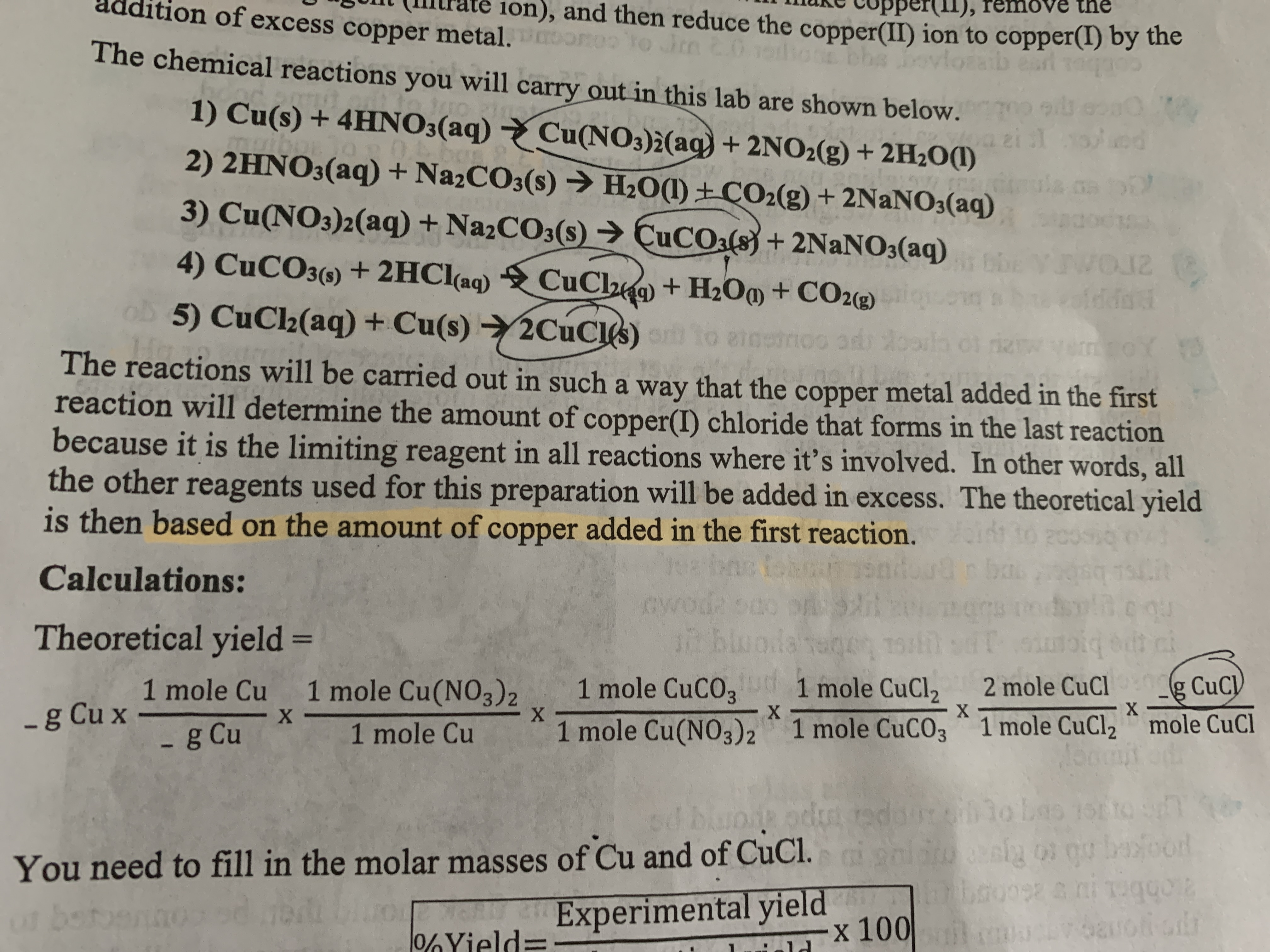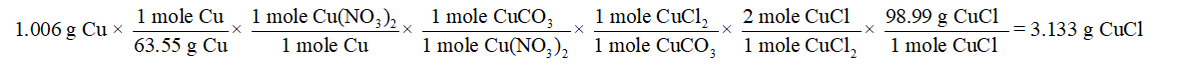# LUnt (IMrate ion), and then reduce the copper(II) ion to copper(I) by thete copper(II), remove theaddition of excess copper metal. ono to novloThe chemical reactions you will carry out in this lab are shown below.1) Cu(s) + 4HNO3(aq) → Cu(NO3)2(aq) + 2NO2(g) + 2H2O(1)2il ed2) 2HNO3(aq) + Na2CO3(s)→ H2O(1) ±ÇO2(g) + 2NaNO3(aq)0.bay3) Cu(NO3)2(aq) + NazCO3(s) → ÇUCO:(s) + 2NANO3(aq)4) CUCO3() + 2HC((aq)CuCh9) + H2O@ + CO2«g)125) CuC2(aq) + Cu(s) 2CUCI)BEPpeto anstmoo adiThe reactions will be carried out in such a way that the copper metal added in the firstreaction will determine the amount of copper(I) chloride that forms in the last reactionbecause it is the limiting reagent in all reactions where it's involved. In other words, allthe other reagents used for this preparation will be added in excess. The theoretical yieldis then based on the amount of copper added in the first reaction.20Calculations:cwoda1n bluodaTheoretical yield =-g CuC)" mole CuCl1 mole Cu 1 mole Cu(NO3)2X.1 mole CuCO31 mole CuCl22 mole CuCl-g Cu x -- g Cu1 mole Cu1 mole Cu(NO3)2 1 mole CuCO3 * 1 mole CuCl2oLas 1stYou need to fill in the molar masses of Cu and of CuCl.Experimental yieldx 100bouos a ni Tuqgo2ucvoaroobor0%Yiel

Question
16 views

Calculate the theoretical yield. My cu is 1.006 ghelp_outlineImage TranscriptioncloseLUnt (IMrate ion), and then reduce the copper(II) ion to copper(I) by the te copper(II), remove the addition of excess copper metal. ono to n ovlo The chemical reactions you will carry out in this lab are shown below. 1) Cu(s) + 4HNO3(aq) → Cu(NO3)2(aq) + 2NO2(g) + 2H2O(1) 2il ed 2) 2HNO3(aq) + Na2CO3(s)→ H2O(1) ±ÇO2(g) + 2NaNO3(aq) 0. bay 3) Cu(NO3)2(aq) + NazCO3(s) → ÇUCO:(s) + 2NANO3(aq) 4) CUCO3() + 2HC((aq) CuCh9) + H2O@ + CO2«g) 12 5) CuC2(aq) + Cu(s) 2CUCI) BEPpe to anstmoo adi The reactions will be carried out in such a way that the copper metal added in the first reaction will determine the amount of copper(I) chloride that forms in the last reaction because it is the limiting reagent in all reactions where it's involved. In other words, all the other reagents used for this preparation will be added in excess. The theoretical yield is then based on the amount of copper added in the first reaction. 20 Calculations: cwoda 1n bluoda Theoretical yield = -g CuC) " mole CuCl 1 mole Cu 1 mole Cu(NO3)2 X. 1 mole CuCO3 1 mole CuCl2 2 mole CuCl -g Cu x - - g Cu 1 mole Cu 1 mole Cu(NO3)2 1 mole CuCO3 * 1 mole CuCl2 oLas 1st You need to fill in the molar masses of Cu and of CuCl. Experimental yield x 100 bouos a ni Tuqgo2 ucvoaroo bor 0%Yiel fullscreen
check_circle

Step 1

In chemistry, there are many ways to check the deviation of experimental data from the accurate value. These different methods provide the difference between accuracy and precisions of the experimental data which can be further used to calculate the error percentage in the experiment.

Step 2

Given:

Cu  =  1.006 g

Molar mass of Cu  =  63.5 g/mol

Molar mass of CuCl = 98.99 g/mol

Step 3

Substitute the values to calculate the theoretical yield:...

### Want to see the full answer?

See Solution

#### Want to see this answer and more?

Solutions are written by subject experts who are available 24/7. Questions are typically answered within 1 hour.*

See Solution
*Response times may vary by subject and question.
Tagged in